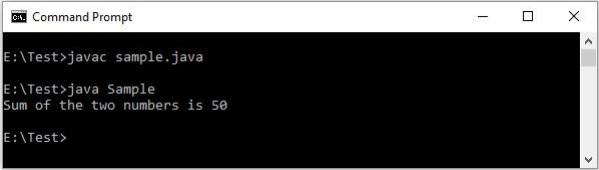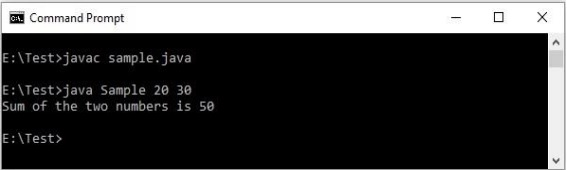# How do you pass a command line argument in Java program?

After writing a Java program you need to compile it using the command javac and run it using the command java. Consider the following code −

public class Sample{
public static void main(String[] args){
int a = 20;
int b = 30;
int c = a+b;
System.out.println("Sum of the two numbers is "+c);
}
}

You can compile and run it from the command line as shown below −Here, we are hard coding (fixing to a specific value) the values of a and b which is not recommendable. To handle this, you can accept parameters from the user using classes from I/O package or Scanner.

Another way is to use command line arguments.

## Command line arguments

The main method accepts an array of values of the type String Java from the user.

public class{
public static void main(String[] args){
}
}

You can pass them at the time of execution right after the class name separated with spaces as −

java ClassName 10 20 30

## Example

In the following Java program, we are accepting two integer values from the command line. And, we are extracting these two from the String array in the main method and converting them to integer −

public class Sample{
public static void main(String[] args){
int a = Integer.parseInt(args);
int b = Integer.parseInt(args);
int c = a+b;
System.out.println("Sum of the two numbers is "+c);
}
}

## Output

You can compile and, run the program by passing the values at execution line through command prompt as shown below −